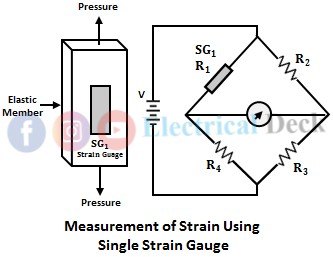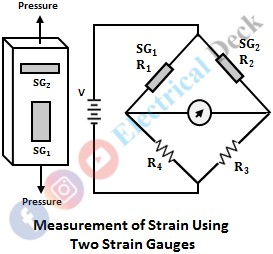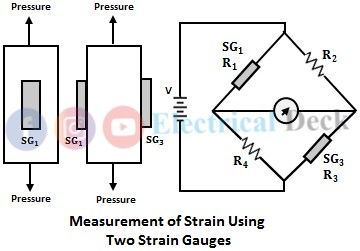# Measurement of Strain with Strain Gauge & Gauge Sensitivity

## What is a Strain Gauge?

The strain gauge is the most extensively used electrical transducer for measuring surface strain. It is a device that works on Piezo-resistive Effect i.e., the tension (or) compression experienced by an object causes to change the resistance of the strain gauge. The change in resistance is the function of change in strain due to tension and compression.

## Working Principle of Strain Gauge :

The principle of strain gauge is based on the change in resistance. We know that the change in length and cross-sectional area of the conductor causes to change in its resistance.

Thus when a strain gauge made up of metal conductor is subjected to strain due to deformation of the object on which it is bonded. There will be a change in resistance of the conductor owing to a change in length and cross-sectional area. This property is called the piezo-resistive effect and so the strain gauges are also called the piezo-resistive strain gauges.

By connecting strain gauge in a balanced Wheatstone bridge. The change in resistance can be measured. The deflection of the meter connected to the bridge gives the value of resistance. The measure of resistance determines the strain applied.

## Measurement of Strain Using Strain Gauge :

#### The strain gauge can be used in different possible arrangements for measuring strain. They are,In this first arrangement, a single strain gauge SG1 is fixed on the elastic member (which is under test) as shown below. Here the strain gauge measures the axial strain in the elastic element. When the strain is applied there will be a change in the resistance of the gauge. These changes in resistance can be measured with the help of the Wheatstone bridge and applied strain is determined. This arrangement is not compensated for changes in temperature.In the other method two active strain gauges, SG1 and SG2 are used to measure strain as shown below. In this arrangement, SG1 and SG2 are placed at right angles to each other. This arrangement is also known as Poisson's arrangement. When strain is applied, the SG1 experiences axial tensile strain, and SG2 experiences transverse compressive strain.The compressive strain is v times the tensile strength. Since the two gauges experience the strain of opposite nature the signal enhancement factor will be (1+v), where v is the Poisson's ratio. This arrangement is compensated for temperature. As the variations in temperature identically affect the two gauges, the set output will be zero. (adsbygoogle = window.adsbygoogle || []).push({}); In another arrangement, two strain gauges are placed such that both will experience an equal amount of axial tensile strains. To measure resistance changes in the gauges due to axial tensile strains, the gauges are connected in any two opposite arms of the bridge as shown below. This arrangement requires two dummy strain gauges to compensate for changes in temperature.Strain can also be measured by employing four active strain gauges. In this arrangement, gauges SG2 and SG4 are placed at right angles to gauges SG1 and SG3. This arrangement compensates for the changes in temperature and its signal enhancement factor is 2(1+v).One of the most common configurations used in pressure, force, displacement, and acceleration transducers is the cantilever beam to which four strain gauges are employed. A measuring system with four active arms as shown in the figure below can be advantageously employed to resolve the components of forces acting on a specimen.

Gauges R1 and R3 are subjected to tension while R2 and R4 are subjected to compression for the load F. If the specimen is subjected to usual loading as indicated by F, as well as a load in the perpendicular direction as indicated by N, the output voltage of the bridge is proportional to the bending only since the load N causes all the four gauges to have identical strain change in resistance with the same sign.

## Gauge Sensitivity :

If a wire or conductor is stretched or compressed, the resistance of the conductor changes because of dimensional changes of length and cross-sectional area. The ratio of change in length to actual length i.e., ΔL/L will give the strain.

The ratio of change in the resistance to the actual resistance i.e., ΔR/R will give the unit change in resistance. Therefore, the gauge sensitivity is described in terms of characteristics called the gauge factor which is defined as the unit change in resistance per unit change in length.

## Applications of Strain Gauges :

#### These are used widely for a variety of electrical transducer devices, mainly because of high accuracy and excellent reliability.Strain gauge transducers are extensively used for analyzing the dynamic strain in complex structures, such as the stress, and strain in bridges where the output of the bridge can be recorded with the help of an oscillograph calibrated to read the instantaneous stress or strains.Strain gauge transducers are used for measuring tension, torque, force, stresses particularly in wind tunnel measurements.Strain gauge transducers are also used for the measurement of force by the strain produced in load rings.

Do not enter any spam links and messages